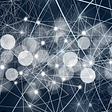# Information theory

The Shannon information content is the amount of information gained when an event x occurs. Mathematically, it is defined as

For example, if a coin shows heads all the time, the events are predictable and we know nothing new. Therefore, the information content is zero. If the coin is unbiased, the probability distribution is uniform and is most unpredictable on whether it will be head or tail next. Therefore, the information content is the highest. Take a second to digest it. In particular, uniform distribution leads to the highest content.

In computer science, the information content can also be viewed as the number of bits to encode the information most efficiently. Utilizing the event frequency, the optimal encoding scheme will be`0b0` for head and `0b1`for tail in a fair coin (or vice versa). For our discussion, we use base 2 for the log. Therefore, the information content for a fair coin flipping is -log₂(½) = 1. i.e. we gain 1-bit of information when we flip the coin once.

X is called a random variable if it holds a value derived from a random process (a stochastic process), e.g. the face value in rolling dice or the total number of heads after flipping a coin 20 times.

The value of X can be modeled with a distribution p(X). For example, let X holds the total sum of rolling ten dies. We can model X with a Gaussian distribution (according to the Central limit theorem).

# Entropy

Entropy H measures the expected information content of a random variable. To calculate the value, we sum up all information content with a weight equal to its occurrence frequency.

For example, in flipping a fair coin, P(X=head)=½ and P(X=tail)=½. Its entropy equals

The average content is one bit. That is, we need an average of one bit to encode an event.

Entropy establishes a lower bound for the average bits to encode events with the probability distribution P.

# Cross-entropy

Cross-entropy H(P, Q) measures the expected number of bits to encode X with distribution P using an encoding scheme targeted for distribution Q.

In ML, we want our predictions Q to match the ground truth P. If they match, the cross-entropy will be the minimum and therefore, we often use it as our training objective.

The cross-entropy for our example is

As shown above, the cost function for many classification problems is simply

# KL-divergence

KL-divergence measures the difference between two distributions P and Q.

It is easy to prove that cross-entropy, entropy and KL Divergence are related:

i.e., KL-Divergence measures the inefficiency of representing P with encoding scheme Q — the extra-bits to encode the information with the sub-optimal scheme. Therefore, KL-divergence is always greater or equal to zero.

Since entropy does not change regardless of how we model it, optimizing KL divergence w.r.t. model parameters is the same as optimizing cross-entropy.

There are a few properties of the KL-divergence that need to be aware of.

KL(p, q) ≥ 0

KL(p, p)=0, and

KL(p, q) ≠KL(q, p) (non-symmetry)

KL(q, p) is called the reverse KL-divergence. The non-symmetry property of KL-divergence has some very significant implications. For example, let’s say the ground truth p is a bimodal distribution (the blue curve below) and we want to model it with a single-mode Gaussian distribution (the red curve). If KL-divergence KL(p, q) is used as the training objective function, we will get a Gaussian distribution that overlaps both modes of the ground truth p that peaks at the trough between the two modes (Diagram a). If the reverse KL-divergence KL(q, p) is used, we will either get one of the local optimal in Diagram b or c.

For KL(p, q), we want q(x) to be non-zero if p(x) is non-zero. Otherwise, if q(x) is small, the KL value will be high. Therefore, it tries to cover what p will cover. For KL(q, p), if P(x) is zero, we want Q(x) to be zero also.

Let’s view the difference in a 2D space. Reverse KL will only cover one of the modes in a bimodal ground truth but the peak of Q will be close to the peak of one of the modes.

The root of the problem is we are using a simple model for complex ground truth. If both models have similar complexity, this will be a non-issue.

# Conditional Entropy

The conditional entropy H(Y|X) is the entropy of Y given X is known. If Y can be separated according to X, this is the weighted entropy of the separated groups and calculated as:

# Information Gain

Mutual information (or Information gain) I(X; Y) is the information obtained on the random variable X when Y is observed. However, the mathematical definition below does not sound intuitive.

But it will be easier with entropy

Intuitively, mutual information measures how much information do we gain by knowing X? If knowing Y gives us all the information about X, the conditional entropy H(X|Y) is zero because there is no more information we needed on X. The mutual information I becomes H(X) (or H(Y)). On the other extreme, if knowing Y gives us no information about X, the conditional entropy is H(X) and the mutual information is zero.

For example, if we know the label (Y) of an object, we gain a lot of information about its raw image (X). We should not mistake its picture with other objects. Therefore the information gain I(X;Y) is high. Let’s visualize this with sets. The mutual information is its overlap.

If random variables X and Y are unrelated, their intersection is empty, and therefore, the mutual information is zero. If random variables X and Y are the same, H(X)=H(Y)=I(X;Y), knowing Y is the same as knowing X.

Let’s go through some of its applications briefly. In a decision tree, we choose a condition to maximize I — a condition that gains the maximum information by separating data according to the branching condition. In another example, InfoGAN maximizes the mutual information between the generated image G and its intended label c. This encourages the GAN model to generate images related to its intended label. The information gain will be used as part of the training objective.

--

--

--

## More from Jonathan Hui

Deep Learning

Love podcasts or audiobooks? Learn on the go with our new app.

## Denoising Documents with background noise.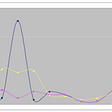## Deep Learning with CIFAR-10 Image Classification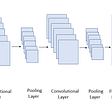## Ways to get started in Machine learning## Cross-entropy for classification## Pooling- Data Consolidation Technique## A Brief Guide To Finding Missing Data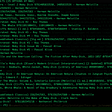## PaddleOCR: The latest lightweight OCR system## What’s the difference between Machine learning algorithms and modelsDeep Learning

## Normal Distributions in Machine Learning## The State of Machine Learning in 8 Papers—February, 2022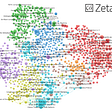## Creating AI Art? Has your Super-SloMo model stopped working?## (A piece of) Machine Learning revolution in practice